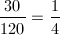# PSAT Math : How to find the probability of an outcome

## Example Questions

### Example Question #42 : Probability

A coporation is deciding who should sit on its leadership committee. If twenty people have applied, and the corporation will choose six applicants to sit on the committee, how many committees are possible?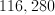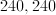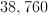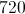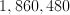Explanation:

This question requires us to make use of the combination formula. In general, if we have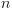total objects or things to choose from, the number of different groups of sizethat we can make is equal to the following: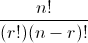where the ! symbol denotes a factorial. In general,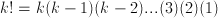.

For example,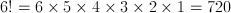.

We have 20 people from whom to select, and each group is going to have 6 people.  Therefore, we will let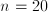and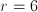.

number of combinations =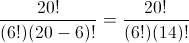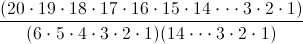=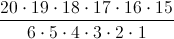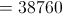The answer is.

### Example Question #141 : Statistics

What is the probability that two dice rolled will add up to 5?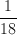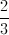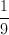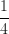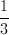Explanation:

There are 6 probabilities for each dice and there are 2 dice. So, you would multiply the individual probabilities together and get 36 possible outcomes.

In order for 2 dice to add to a sum of 5, you would need to roll:

1 4; 2 3; 3 2; 4 1 [each dice can roll as either number]

That is a total of 4 outcomes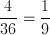### Example Question #1492 : Psat Mathematics

A bag containing only red, green and blue marbles has 10 red, 20 green, and 20 blue marbles.  If Laura reaches in and removes a single marble at random, what is the probability she draws a green marble?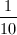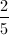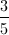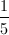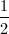Explanation:

Probability = the number of desired outcomes divided by the number of total possible outsomes.  20 marbles are green out of a total of 50.  Therefore: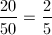### Example Question #41 : Probability

A deck of 52 cards is divided equally into four suits: hearts, diamonds, clubs and spades, and two cards are drawn at random.  The first card drawn is a heart. What is the probability that the second card drawn is also a heart?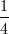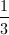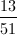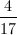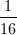Explanation:

There are 13 hearts in the deck of 52 cards.  The first card is already assumed to be a heart, but by removing it the numbers change.  When drawing the second card, there are now 12 hearts out of 51 total cards.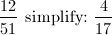### Example Question #51 : How To Find The Probability Of An Outcome

A game is played where players take turns rolling two six-sided dice, each with sides numbered 1-6.  The numbers on the two dice are added together for each player's score.  If the first player rolls a sum of 10, what is the probability that the second player rolls a HIGHER total?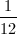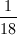Explanation:

A roll of two 6-sided dice yields 36 total possible outcomes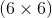. There are three possible ways to roll a sum higher than 10:

5, 6

6, 5

6, 6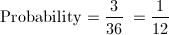### Example Question #3131 : Sat Mathematics

10 cards, each with a distinct number from 1-10, are placed face-down on a table.  If Matt chooses a card at random, what is the probability his card will display a prime number?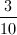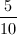Explanation:

Total outcomes: 10

Desired outcomes (prime numbers) = 4   (2, 3, 5, 7)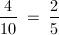### Example Question #51 : Probability

A coin with two sides, heads and tails, is flipped repeatedly and the result recorded. Assuming an equal chance of it landing either way, what is the probability of each of the first four flips being heads?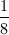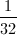Explanation: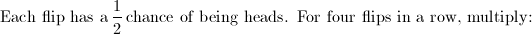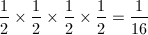### Example Question #3132 : Sat Mathematics

A coin with two sides, heads and tails, is flipped 10 times and the results recorded.  What is the probability that the 10th flip results in tails?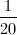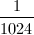Explanation: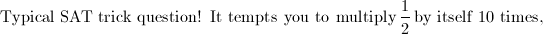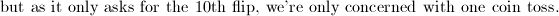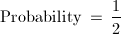### Example Question #52 : Probability

A dresser drawer contains only 4 blue socks, 6 white socks, and 2 red socks.  If two socks are pulled from the drawer at random, what is the probability that they are both white?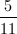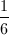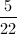Explanation:

For this question to be satsified, both socks drawn must be white.

First draw:  6 white socks out of 12 total: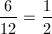Second draw: 5 white socks out of 11 total:Multiply together to find the answer: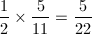### Example Question #51 : How To Find The Probability Of An Outcome

In a hotel with 120 rooms, 30 of them have red carpet.  A hotel manager has lost his keys somewhere in the hotel.  If there is an equal chance of the keys being in any room, what is the probability that they are in a room with red carpet?